Chin. Phys. B, 2020, Vol. 29(11): 110504    DOI: 10.1088/1674-1056/abbbfe
 GENERAL Prev   Next

# Memristor-based hyper-chaotic circuit for image encryption

Jiao-Jiao Chen(陈娇娇)1,2, Deng-Wei Yan(闫登卫)1,2, Shu-Kai Duan(段书凯)1,2,3,4,5,6, Li-Dan Wang(王丽丹)1,2,3,4,5,†()
1 College of Electronic and Information Engineering, Southwest University, Chongqing 400715, China
2 Chongqing Key Laboratory of Nonlinear Circuits and Intelligent Information Processing, Southwest University, Chongqing 400715, China
3 Intelligent Transmission and Control Technology Joint Engineering Laboratory, Chongqing 400715, China
4 Brain-inspired Computing and Intelligent Control Chongqing Key Laboratory, Chongqing 400715, China
5 Chongqing Collaborative Innovation Center for Brain Science, Chongqing 400715, China
6 School of Artificial Intelligence, Southwest University, Chongqing 400715, China
 Abstract  The memristor is a kind of non-linear element with memory function, which can be applied to chaotic systems to increase signal randomness and complexity. In this paper, a new four-dimensional hyper-chaotic system is designed based on a flux controlled memristor model, which can generate complex chaotic attractors. The basic dynamic theory analysis and numerical simulations of the system, such as the stability of equilibrium points, the Lyapunov exponents and dimension, Poincare maps, the power spectrum, and the waveform graph prove that it has rich dynamic behaviors. Then, the circuit implementation of this system is established. The consistency of simulation program with integrated circuit emphasis (SPICE) simulation and numerical analysis proves the ability to generate chaos. Finally, a new image encryption scheme is designed by using the memristor-based hyper-chaotic system proposed in this paper. The scheme involves a total of two encryptions. By using different security analysis factors, the proposed algorithm is compared with other image encryption schemes, including correlation analysis, information entropy, etc. The results show that the proposed image encryption scheme has a large key space and presents a better encryption effect. Keywords:  memristor      SPICE simulation      hyper-chaotic system      image encryption Received:  30 April 2020      Revised:  04 September 2020      Published:  03 November 2020 Fund: the National Key Research and Development Program of China (Grant No. 2018YFB1306600), the National Natural Science Foundation of China (Grant Nos. 62076207 and 62076208), and the Fundamental Science and Advanced Technology Research Foundation of Chongqing, China (Grant Nos. cstc2017jcyjBX0050). Corresponding Authors:  †Corresponding author. E-mail: ldwang@swu.edu.cn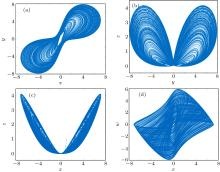Fig. 1.  Phase portraits of system (1) with a = 3, b = 1, c = 9, e = 0.2, m = 1 in (a) x–y plane, (b) y–z plane, (c) x–z plane, and (d) x–w plane.Table 1.  Equilibrium points of formula (5) when x < C5.Table 2.  Equilibrium points of formula (4) when C5 ≤ x ≤ C6.Table 3.  Equilibrium points of formula (5) when x > C6.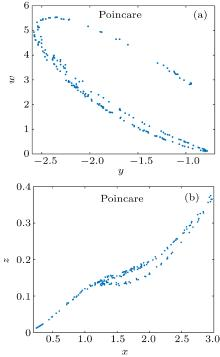Fig. 2.  Poincare map of (a) x = 0 and (b) y = 0.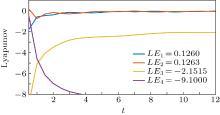Fig. 3.  Lyapunov exponents of the system with a = 3, b = 1, c = 9, e = 0.2, m = 1.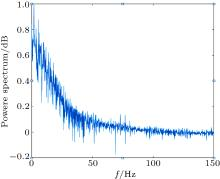Fig. 4.  Power spectrum of the system.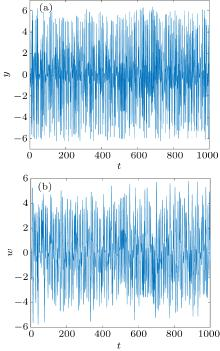Fig. 5.  Time domain waveform of (a) t–y and (b) t–w.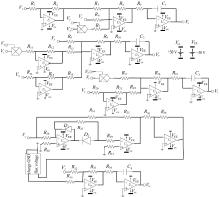Fig. 6.  Hardware circuit implementation of new hyper-chaotic system.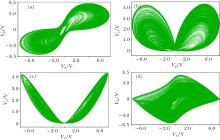Fig. 7.  SPICE simulation results in (a) x–y, (b) y–z, (c) x–z, and (d) x–w planes of the system.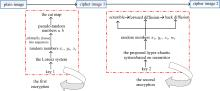Fig. 8.  Block diagram of proposed image encryption.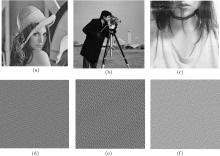Fig. 9.  Plain image of (a) Lena, (b) Cameraman, and (c) Girl; first encrypted image of (d) Lena, (e) Cameraman, and (f) Girl.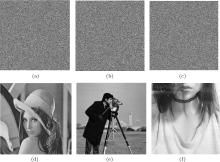Fig. 10.  Second encrypted image of (a) Lena, (b) Cameraman, and (c) Girl; decrypted image of (d) Lena, (e) Cameraman, and (f) Girl.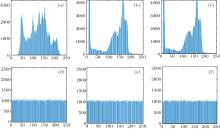Fig. 11.  Gray histogram of plaintext of (a) Lena, (b) Cameraman, (c) Girl; gray histogram of ciphered image of (d) Lena, (e) Cameraman, and (f) Girl.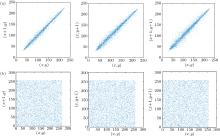Fig. 12.  Correlation distribution of (a) plaintext and (b) ciphertext image in horizontal, vertical, and diagonal direction of Lena.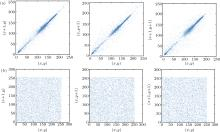Fig. 13.  Correlation distribution of (a) plaintext and (b) ciphertext image in horizontal, vertical, and diagonal direction of Cameraman.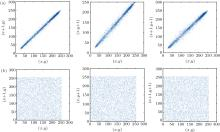Fig. 14.  Correlation distribution of (a) plaintext and (b) ciphertext image in horizontal, vertical, and diagonal direction of Girl.Table 4.  Correlation coefficients of plain image and cipher image.Table 5.  Information entropy of test images.Table 6.  Values of NPCR, UACI, and BACI of different images after modifying one pixel value.